# How to add text or character to every cell in Excel

Wondering how to add text to an existing cell in Excel? In this article, you will learn a few really simple ways to insert characters in any position in a cell.

When working with text data in Excel, you may sometimes need to add the same text to existing cells to make things clearer. For example, you might want to put some prefix at the beginning of each cell, insert a special symbol at the end, or place certain text before a formula.

I guess everyone knows how to do this manually. This tutorial will teach you how to quickly add strings to multiple cells using formulas and automate the work with VBA or a special Add Text tool.

## Excel formulas to add text/character to cell

To add a specific character or text to an Excel cell, simply concatenate a string and a cell reference by using one of the following methods.

### Concatenation operator

The easiest way to add a text string to a cell is to use an ampersand character (&), which is the concatenation operator in Excel.

"text"&cell

This works in all versions of Excel 2007 - Excel 365.

### CONCATENATE function

The same result can be achieved with the help of the CONCATENATE function:

CONCATENATE("text", cell)

The function is available in Excel for Microsoft 365, Excel 2019 - 2007.

### CONCAT function

To add text to cells in Excel 365, Excel 2019, and Excel Online, you can use the CONCAT function, which is a modern replacement of CONCATENATE:

CONCAT("text", cell)
Note. Please pay attention that, in all formulas, text should be enclosed in quotation marks.

These are the general approaches, and the below examples show how to apply them in practice.

## How to add text to the beginning of cells

To add certain text or character to the beginning of a cell, here's what you need to do:

1. In the cell where you want to output the result, type the equals sign (=).
2. Type the desired text inside the quotation marks.
3. Type an ampersand symbol (&).
4. Select the cell to which the text shall be added, and press Enter.

Alternatively, you can supply your text string and cell reference as input parameters to the CONCATENATE or CONCAT function.

For example, to prepend the text "Project:" to a project name in A2, any of the below formulas will work.

In all Excel versions:

`="Project:"&A2`

`=CONCATENATE("Project:", A2)`

In Excel 365 and Excel 2019:

`=CONCAT("Project:", A2)`

Enter the formula in B2, drag it down the column, and you will have the same text inserted in all cells.

Tip. The above formulas join two strings without spaces. To separate values with a whitespace, type a space character at the end of the prepended text (e.g. "Project: ").For convenience, you can input the target text in a predefined cell (E2) and add two text cells together:

Without spaces:

`=\$E\$2&A2`

`=CONCATENATE(\$E\$2, A2)`

With spaces:

`=\$E\$2&" "&A2`

`=CONCATENATE(\$E\$2, " ", A2)`

Please notice that the address of the cell containing the prepended text is locked with the \$ sign, so that it won't shift when copying the formula down.

With this approach, you can easily change the added text in one place, without having to update every formula.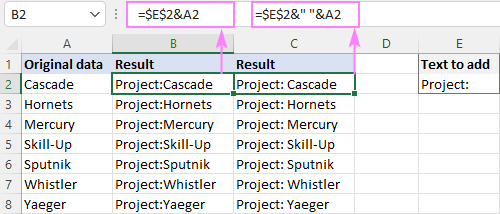## How to add text to the end of cells in Excel

To append text or specific character to an existing cell, make use of the concatenation method again. The difference is in the order of the concatenated values: a cell reference is followed by a text string.

For instance, to add the string "-US" to the end of cell A2, these are the formulas to use:

`=A2&"-US"`

`=CONCATENATE(A2, "-US")`

`=CONCAT(A2, "-US")`

Alternatively, you can enter the text in some cell, and then join two cells with text together:

`=A2&\$D\$2`

`=CONCATENATE(A2, \$D\$2)`

Please remember to use an absolute reference for the appended text (\$D\$2) for the formula to copy correctly across the column.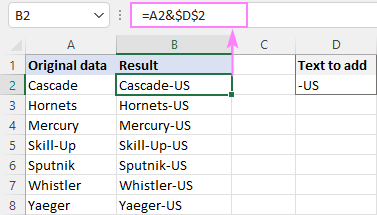## Add characters to beginning and end of a string

Knowing how to prepend and append text to an existing cell, there is nothing that would prevent you from using both techniques within one formula.

As an example, let's add the string "Project:" to the beginning and "-US" to the end of the existing text in A2.

`="Project:"&A2&"-US"`

`=CONCATENATE("Project:", A2, "-US")`

`=CONCAT("Project:", A2, "-US")`

With the strings input in separate cells, this works equally well: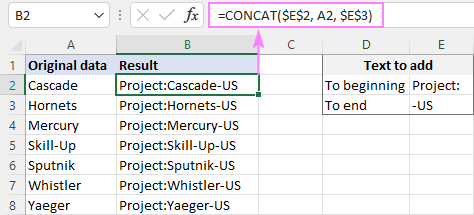## Combine text from two or more cells

To place values from multiple cells into one cell, concatenate the original cells by using the already familiar techniques: an ampersand symbol, CONCATENATE or CONCAT function.

For example, to combine values from columns A and B using a comma and a space (", ") for the delimiter, enter one of the below formulas in B2, and then drag it down the column.

Add text from two cells with an ampersand:

`=A2&", "&B2`

Combine text from two cells with CONCAT or CONCATENATE:

`=CONCATENATE(A2, ", ", B2)`

`=CONCAT(A2, ", ", B2)`

When adding text from two columns, be sure to use relative cell references (like A2), so they adjust correctly for each row where the formula is copied.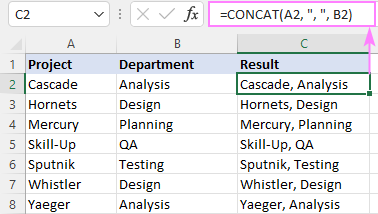To combine text from multiple cells in Excel 365 and Excel 2019, you can leverage the TEXTJOIN function. Its syntax provides for a delimiter (the first argument), which makes the formular more compact and easier to manage.

For example, to add strings from three columns (A, B and C), separating the values with a comma and a space, the formula is:

`=TEXTJOIN(", ", TRUE, A2, B2, C2)`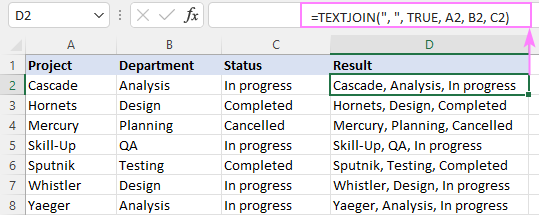## How to add special character to cell in Excel

To insert a special character in an Excel cell, you need to know its code in the ASCII system. Once the code is established, supply it to the CHAR function to return a corresponding character. The CHAR function accepts any number from 1 to 255. A list of printable character codes (values from 32 to 255) can be found here.

To add a special character to an existing value or a formula result, you can apply any concatenation method that you like best.

For example, to add the trademark symbol (™) to text in A2, any of the following formulas will work:

`=A2&CHAR(153)`

`=CONCATENATE(A2&CHAR(153))`

`=CONCAT(A2&CHAR(153))`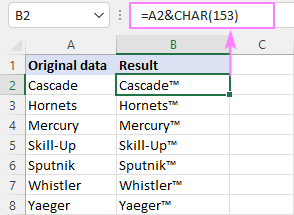## How to add text to formula in Excel

To add a certain character or text to a formula result, just concatenate a string with the formula itself.

Let's say, you are using this formula to return the current time:

`=TEXT(NOW(), "h:mm AM/PM")`

To explain to your users what time that is, you can place some text before and/or after the formula.

Insert text before formula:

`="Current time: "&TEXT(NOW(), "h:mm AM/PM")`

`=CONCATENATE("Current time: ", TEXT(NOW(), "h:mm AM/PM"))`

`=CONCAT("Current time: ", TEXT(NOW(), "h:mm AM/PM"))`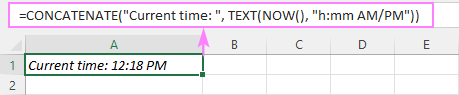`=TEXT(NOW(), "h:mm AM/PM")&" - current time"`

`=CONCATENATE(TEXT(NOW(), "h:mm AM/PM"), " - current time")`

`=CONCAT(TEXT(NOW(), "h:mm AM/PM"), " - current time")`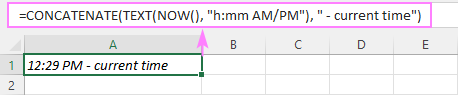Add text to formula on both sides:

`="It's " &TEXT(NOW(), "h:mm AM/PM")& " here in Gomel"`

`=CONCATENATE("It's ", TEXT(NOW(), "h:mm AM/PM"), " here in Gomel")`

`=CONCAT("It's ", TEXT(NOW(), "h:mm AM/PM"), " here in Gomel")`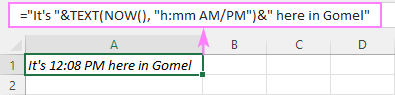## How to insert text after Nth character

To add a certain text or character at a certain position in a cell, you need to split the original string into two parts and place the text in between. Here's how:

1. Extract a substring preceding the inserted text with the help of the LEFT function:
LEFT(cell, n)
2. Extract a substring following the text using the combination of RIGHT and LEN:
RIGHT(cell, LEN(cell) -n)
3. Concatenate the two substrings and the text/character using an ampersand symbol.

The complete formula takes this form:

LEFT(cell, n) & "text" & RIGHT(cell, LEN(cell) -n)

The same parts can be joined together by using the CONCATENATE or CONCAT function:

CONCATENATE(LEFT(cell, n), "text", RIGHT(cell, LEN(cell) -n))

The task can also be accomplished by using the REPLACE function:

REPLACE(cell, n+1, 0, "text")

The trick is that the num_chars argument that defines how many characters to replace is set to 0, so the formula actually inserts text at the specified position in a cell without replacing anything. The position (start_num argument) is calculated using this expression: n+1. We add 1 to the position of the nth character because the text should be inserted after it.

For example, to insert a hyphen (-) after the 2nd character in A2, the formula in B2 is:

`=LEFT(A2, 2) &"-"& RIGHT(A2, LEN(A2) -2)`

Or

`=CONCATENATE(LEFT(A2, 2), "-", RIGHT(A2, LEN(A2) -2))`

Or

`=REPLACE(A2, 2+1, 0, "-")`

Drag the formula down, and you will have the same character inserted in all the cells: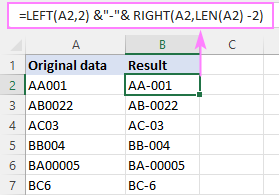## How to add text before/after a specific character

To insert certain text before or after a particular character, you need to determine the position of that character in a string. This can be done with the help of the SEARCH function:

SEARCH("char", cell)

Once the position is determined, you can add a string exactly at that place by using the approaches discussed in the above example.

### Add text after specific character

To insert some text after a given character, the generic formula is:

LEFT(cell, SEARCH("char", cell)) & "text" & RIGHT(cell, LEN(cell) - SEARCH("char", cell))

Or

CONCATENATE(LEFT(cell, SEARCH("char", cell)), "text", RIGHT(cell, LEN(cell) - SEARCH("char", cell)))

For instance, to insert the text (US) after a hyphen in A2, the formula is:

`=LEFT(A2, SEARCH("-", A2)) &"(US)"& RIGHT(A2, LEN(A2) - SEARCH("-", A2))`

Or

`=CONCATENATE(LEFT(A2, SEARCH("-", A2)), "(US)", RIGHT(A2, LEN(A2) -SEARCH("-", A2)))`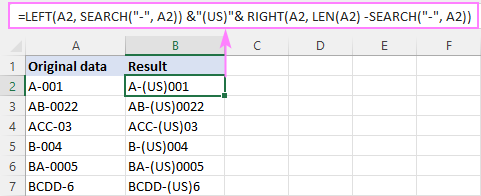### Insert text before specific character

To add some text before a certain character, the formula is:

LEFT(cell, SEARCH("char", cell) -1) & "text" & RIGHT(cell, LEN(cell) - SEARCH("char", cell) +1)

Or

CONCATENATE(LEFT(cell, SEARCH("char", cell) - 1), "text", RIGHT(cell, LEN(cell) - SEARCH("char", cell) +1))

As you see, the formulas are very similar to those that insert text after a character. The difference is that we subtract 1 from the result of the first SEARCH to force the LEFT function to leave out the character after which the text is added. To the result of the second SEARCH, we add 1, so that the RIGHT function will fetch that character.

For example, to place the text (US) before a hyphen in A2, this is the formula to use:

`=LEFT(A2, SEARCH("-", A2) -1) &"(US)"& RIGHT(A2, LEN(A2) -SEARCH("-", A2) +1)`

Or

`=CONCATENATE(LEFT(A2, SEARCH("-", A2) -1), "(US)", RIGHT(A2, LEN(A2) -SEARCH("-", A2) +1))`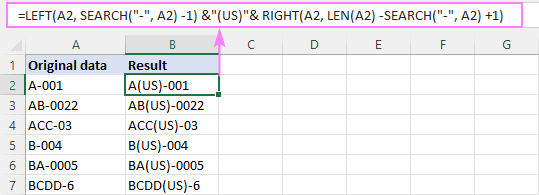Notes:

• If the original cell contains multiple occurrences of a character, the text will be inserted before/after the first occurrence.
• The SEARCH function is case-insensitive and cannot distinguish lowercase and uppercase characters. If you aim to add text before/after a lowercase or uppercase letter, then use the case-sensitive FIND function to locate that letter.

## How to add space between text in Excel cell

In fact, it is just a specific case of the two previous examples.

To add space at the same position in all cells, use the formula to insert text after nth character, where text is the space character (" ").

For example, to insert a space after the 10th character in cells A2:A7, enter the below formula in B2 and drag it through B7:

`=LEFT(A2, 10) &" "& RIGHT(A2, LEN(A2) -10)`

Or

`=CONCATENATE(LEFT(A2, 10), " ", RIGHT(A2, LEN(A2) -10))`

In all the original cells, the 10th character is a colon (:), so a space is inserted exactly where we need it: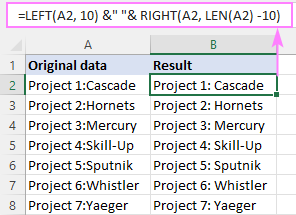To insert space at a different position in each cell, adjust the formula that adds text before/after a specific character.

In the sample table below, a colon (:) is positioned after the project number, which may contain a variable number of characters. As we wish to add a space after the colon, we locate its position using the SEARCH function:

`=LEFT(A2, SEARCH(":", A2)) &" "& RIGHT(A2, LEN(A2)-SEARCH(":", A2))`

Or

`=CONCATENATE(LEFT(A2, SEARCH(":", A2)), " ", RIGHT(A2, LEN(A2)-SEARCH(":", A2)))`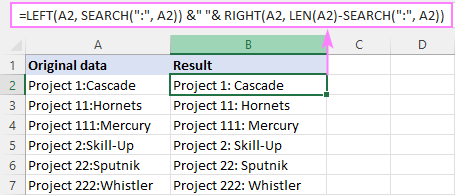## How to add the same text to existing cells with VBA

If you often need to insert the same text in multiple cells, you can automate the task with VBA.

### Prepend text to beginning

The below macros add text or a specific character to the beginning of all selected cells. Both codes rely on the same logic: check each cell in the selected range and if the cell is not empty, prepend the specified text. The difference is where the result is placed: the first code makes changes to the original data while the second one places the results in a column to the right of the selected range.

If you have little experience with VBA, this step-by-step guide will walk you through the process: How to insert and run VBA code in Excel.

Macro 1: adds text to the original cells

```Sub PrependText()
Dim cell As Range

For Each cell In Application.Selection
If cell.Value <> "" Then cell.Value = "PR-" & cell.Value
Next
End Sub
```

This code inserts the substring "PR-" to the left of an existing text. Before using the code in your worksheet, be sure to replace our sample text with the one you really need.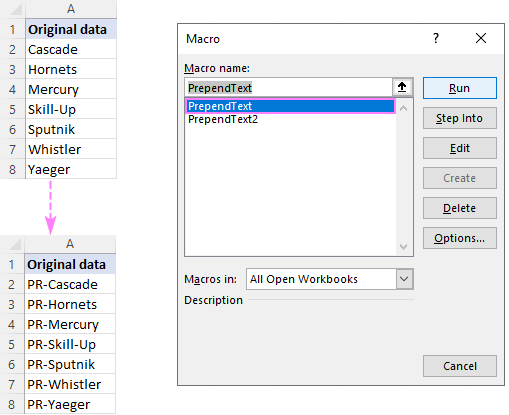Macro 2: places the results in the adjacent column

```Sub PrependText2()
Dim cell As Range

For Each cell In Application.Selection
If cell.Value <> "" Then cell.Offset(0, 1).Value = "PR-" & cell.Value
Next
End Sub
```

Before running this macro, make sure there is an empty column to the right of the selected range, otherwise the existing data will be overwritten.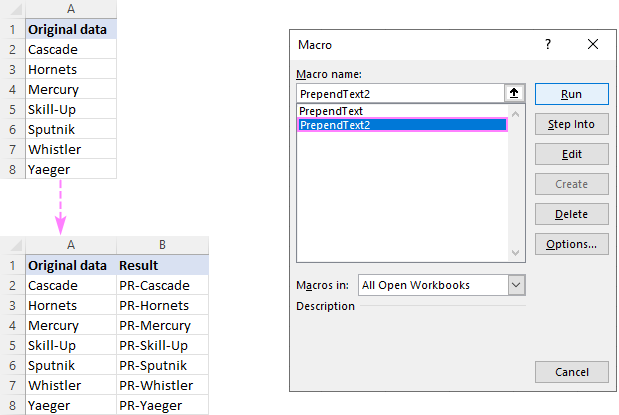### Append text to end

If you are looking to add a specific string/character to the end of all selected cells, these codes will help you get the work done quickly.

Macro 1: appends text to the original cells

```Sub AppendText()
Dim cell As Range

For Each cell In Application.Selection
If cell.Value <> "" Then cell.Value = cell.Value & "-PR"
Next
End Sub
```

Our sample code inserts the substring "-PR" to the right of an existing text. Naturally, you can change it to whatever text/character you need.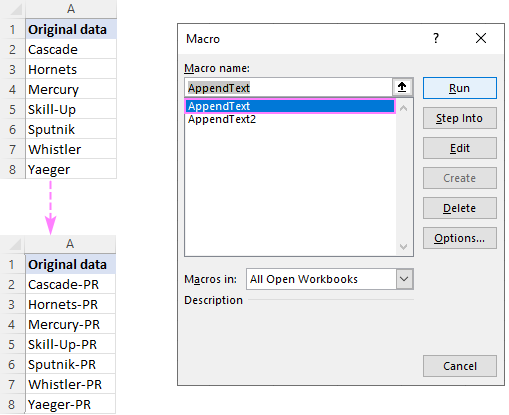Macro 2: places the results in another column

```Sub AppendText2()
Dim cell As Range

For Each cell In Application.Selection
If cell.Value <> "" Then cell.Offset(0, 1).Value = cell.Value & "-PR"
Next
End Sub
```

This code places the results in a neighboring column. So, before you run it, make certain you have at least one empty column to the right of the selected range, otherwise your existing data will be overwritten.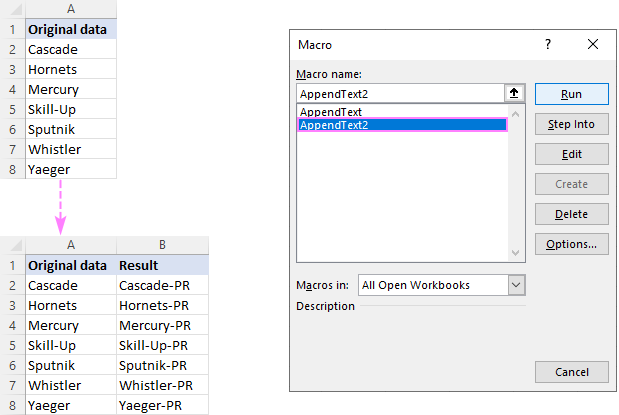## Add text or character to multiple cells with Ultimate Suite

In the first part of this tutorial, you've learned a handful of different formulas to add text to Excel cells. Now, let's me show you how to accomplish the task with a few clicks :)

With Ultimate Suite installed in your Excel, here are the steps to follow:

2. On the Ablebits tab, in the Text group, click Add.
3. On the Add Text pane, type the character/text you wish to add to the selected cells, and specify where it should be inserted:
• At the beginning
• At the end
• Before specific text/character
• After specific text/character
• After Nth character from the beginning or end
4. Click the Add Text button. Done!

As an example, let's insert the string "PR-" after the "-" character in cells A2:A7. For this, we configure the following settings: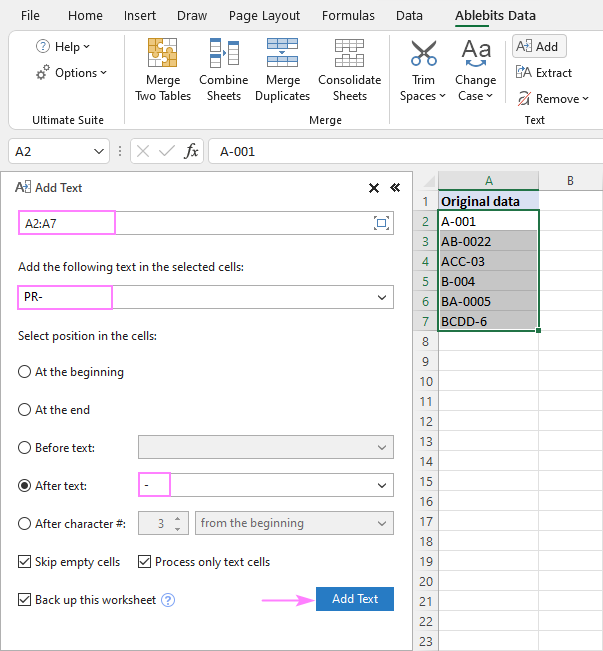A moment later, we get the desired result: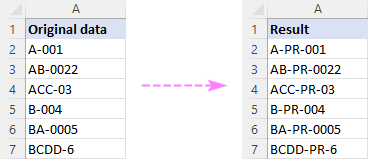These are the best ways to add characters and text strings in Excel. I thank you for reading and hope to see you on our blog next week!

Add text to cell in Excel - formula examples (.xlsm file)
Ultimate Suite - trial version (.zip file)

## You may also be interested in

### 16 comments to "How to add text or character to every cell in Excel"

1.Arghya says:

A B
1000403 DRUGS ALLOPURINOL
(ALLOPURINOL USP)
1000448 TRI ETHYL
ALUMINUM
1000465 TRI ETHYL
ALUMINUM
1000552 DRUGS: LOSARTAN
POTASSIUM USP
(LOSARTANPOTASSIUM)
1000916 COMBED
70%POLYESTER FILAMENT YARN
42%
1001356 BOPP FILMS
BIAXIALLY ORIENTED POLYPROPYLENE FILM

in this database, I want to add texts spread over 2-3-4-n nos of Cells at Cl-B against individual entry(first column) in a single cell. Considering the database is huge and number of cell over which text is spread is not limited, use of concatenate may not be helpful.

•Alexander Trifuntov (Ablebits Team) says:

Hi!
I don't really understand what you want to do. But for merging cells, you might find this article helpful: Merge and combine cells in Excel without losing data.
If this is not what you wanted, please describe the problem in more detail. Write an example of the result you want to get.

2.Chuck Newbanks says:

How can I add text to a formula like =SUM(224/12) so the result would be 18.6 days

3.Elyse says:

How do I add a comma and a space between characters? My cells return multiple characters in one cell (I want that) and I would like to add a comma and space between each character.
Elyse

•Alexander Trifuntov (Ablebits Team) says:

Hello!
What formula do you use to get the characters in a cell? We need to change this formula. To write text with commas in another cell you can use the TEXTJOIN function

=TEXTJOIN(",",TRUE,MID(A1,ROW(A1:A50),1))

4.Sameer says:

I want to add "Address" but can not put as =""Address"" since it does not take it as text. how I can add this ?

•Alexander Trifuntov (Ablebits Team) says:

Hi!
If Excel does not treat your text as a formula, add an apostrophe ' before the text.

5.Ayen Bernas says:

how do you enter name, and letter by letter it goes to each next Cell,
for example : JOHN RAMBO,
J O H N R A M B O in each cell

•Alexander Trifuntov (Ablebits Team) says:

Hi!
After each letter, press the cursor arrow key to move to the next cell.

•Ayen Bernas says:

i need to copy data from other form and put in an excel sheet, which read each letter for each cell, kind of hard adding list of name to it by using arrow keys, as names from my country had kind of long names...

•Alexander Trifuntov (Ablebits Team) says:

Hi!
Sorry, it's not quite clear what you are trying to achieve. Please describe your problem in more detail.

•Ayen Bernas says:

okay, the sheet than i had was make by the printing company and some how if edited it will be printed differently, im suppose to fill in the name from a physical form into the sheet, in which read each letter of the name in each cell, the problem was it become a hassle each time for me to enter the name for example :
JOHN DOE
i had to enter the name
J "ARROW KEY" O "ARROW KEY" H "ARROW KEY" N "ARROW KEY" *BLANK* "ARROW KEY" D "ARROW KEY" O "ARROW KEY" E

so in the sheet it will be [ J ][ O ][ H ][ N ][ ][ D ][ O ][ E ]

its okay to type in like this, but if you had 300 names with 21 letter, well... poop..
it will be helpful if you can solve this silly problem of mine... thank you

•Alexander Trifuntov (Ablebits Team) says:

Hi!
Your problem can be solved with a VBA macro. But now I can only help you with Excel formulas.

6.BASHIR WASAGU says:

Good day.
Please which formula in excel can ad space after 2nd upper case character.. e.g. "JohnDavis" to appear as "John Davis"

Thank you

•Alexander Trifuntov (Ablebits Team) says:

Hello!
To insert a space before the second uppercase letter, use the formula:

=LEFT(A1,MATCH(TRUE,EXACT(MID(A1,ROW(2:50),1), UPPER(MID(A1,ROW(2:50),1))),0))&" "&MID(A1,MATCH(TRUE,EXACT(MID(A1,ROW(2:50),1), UPPER(MID(A1,ROW(2:50),1))),0)+1,50)